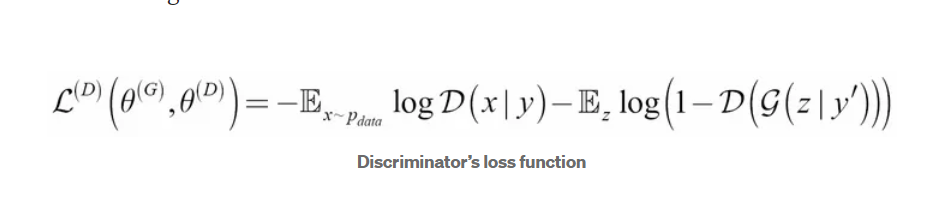top of page
Search

# Computer Vision- interview questions

Updated: Jul 19

What are the drawbacks of R CNN?

1. slow at test, need to run full forward pass for each region.

2. SVM are post hoc, leading to multi level pipeline.

How FastRNN resolved this limitations?

1. image is passed through cnn, not all the proposed regions.

2. end to end training removed multi stage training of RCNN.What is the unique component in Faster RCNN ?

Faster RCNN uses Region proposed Network which eliminated use of external method to generate regions but regions are trained during training.

How to calculate output tensor shape in yolo-v1?

Yolo system models detection as a regression problem. It divides the image into an S × S grid and for each grid cell predicts B bounding boxes, confidence for those boxes, and C class probabilities. These predictions are encoded as an S × S × (B ∗ 5 + C) tensor.

Each bounding box consists of 5 predictions: x, y, w, h, and confidence. The (x, y) coordinates represent the center of the box relative to the bounds of the grid cell. The width and height are predicted relative to the whole image. Finally the confidence prediction represents the IOU between the predicted box and any ground truth box.

What are some self supervised techniques of feature extraction in computer vision task?

1. SimCLR ( Simple Contrasive Learning)

2. Rotnet ( Rotation Net)

3. BYOL

sim_clr
.pdf

rot_net
.pdf

What are popular semantic segmentation techniques?

1. Deeplab

deepLab_atrousConvolution_imageSegmentation
.pdf

2. Unet

unet_image_segmentation
.pdf

what are the properties of Minmax loss. How discriminator & generator both uses it for corresponding optimisation?

GANs, the generator tries to minimize the following function while the discriminator tries to maximize it:In this function:

• D(x) is the discriminator's estimate of the probability that real data instance x is real.

• Ex is the expected value over all real data instances.

• G(z) is the generator's output when given noise z.

• D(G(z)) is the discriminator's estimate of the probability that a fake instance is real.

• Ez is the expected value over all random inputs to the generator (in effect, the expected value over all generated fake instances G(z)).

• The formula derives from the cross-entropy between the real and generated distributions.

The generator can't directly affect the log(D(x)) term in the function, so, for the generator, minimizing the loss is equivalent to minimizing log( 1 - D(G(z))).

What is conditional GAN (cGAN)?

In normal GAN we don't have control over what type ( class) of image is generated. Using cGAN we can conditioned on output image. Here class of image is also passed as input to both discriminator and generator.Where do we use CycleGAN?

This is useful when we want to generate an image but want to put constrain based on input image. Like change only in color of input image, covert horse to zebra only by adding stripes.

What is FGSM?

FGSM ( Fast Gradient Signal method) is used to generate adversarial images. Here small amount is noise is added in input image so that model starts predicting incorrect class.

Noise is added by exploiting loss function. So input image is modified( adding noise) in a way that it will increase the loss for particular image so that incorrect prediction can be generated.Input layer has 2 kernels of size 3*3 and output layer has 3 kernels of size 3*3. How many trainable parameters are there?

Every value of a kernel is trainable weight and 1 bias term for every kernel so input layer would have 2*( 3*3 +1)= 20 parameters.

Next layer would have 2( previous layer channel)*(3*3*3)+3= 2*27+3= 57 parameters.

What is the core idea behind GoogleNet?

it uses 9 blocks of inception block . An inception block consist of various filters of different shapes unlike other state of art models.Error is also propagated through various points in the network, rendering in better learning.